### Home > PC > Chapter 8 > Lesson 8.1.1 > Problem8-7

8-7.
1. Find the following limits. Homework Help ✎

1.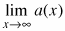, where a(x) = x6

2.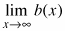, where b(x) = x6 − 12x5 + 7x3 − 400

3.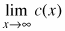, where c(x) = −3x7

4.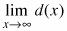, where d(x) = −3x7 + 1000x + 48000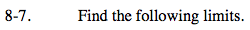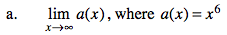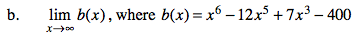When x is extremely large, what is the only term that matters for this limit?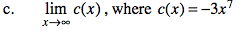−∞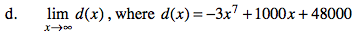When x is extremely large, what is the only term that matters for this limit?